# Data Handling Class 8 Extra Questions Maths Chapter 5

Data Handling Class 8 Extra Questions Maths Chapter 5

### NCERT Solutions for Class 8 Maths Chapter 5 Data Handling Exercise 5.3

Ex 5.3 Class 8 Maths Question 1.
List the outcomes you can see in these experiments.
(i) Spinning a wheel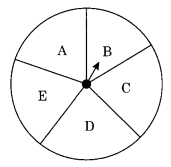(ii) Tossing two coins together
Solution:
(i) On spinning the wheel, we can get the following outcomes B, C, D, E and A.
(ii) When two coins are tossed together, we get the following outcomes
HH, HT, TH, TT (Where H denotes Head and T denotes Tail)

Ex 5.3 Class 8 Maths Question 2.
When a die is thrown, list the outcomes of an event of getting
(i) (a) a prime number
(b) not a prime number
(ii) (a) a number greater than 5
(b) a number not greater than 5
Solution:
(i) (a) The prime number are 2, 3 and 5
Required outcomes = 2, 3 and 5
(b) Outcomes for not a prime number are 1, 4 and 6
Required outcomes = 1, 4, 6.
(ii) (a) Outcomes for a number greater than 5 = 6
Required outcome = 6
(b) Outcomes for a number not greater than 5 are 1, 2, 3, 4, 5
Required outcomes = 1, 2, 3, 4, 5.

Ex 5.3 Class 8 Maths Question 3.
Find the
(i) Probability of the pointer stopping on D in (Question 1-(a))?
(ii) Probability of getting an ace from a well-shuffled deck of 52 playing cards?
(iii) Probability of getting a red apple, (see figure below)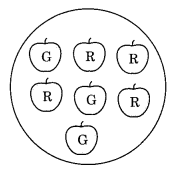Solution:
(i) Refer to fig. Question 1-(a)
Total number of sectors = 5
Number of sector where the pointer stops = 1, i.e. D
Probability of pointer stopping at D = 1/5
(ii) Number of aces = 4 (one from each suit i.e. heart, diamond, club and spade)
Total number of playing cards = 52
Probability of getting an ace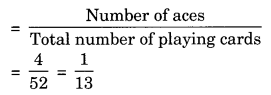(iii) Total number of apples = 7
Number of red apples = 4
Probability of getting red apples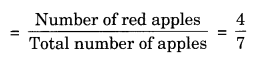Ex 5.3 Class 8 Maths Question 4.
Numbers 1 to 10 are written on ten separate slips (one number on one slip), kept in a box and mixed well. One slip is choosen from the box without looking into it. What is the probability of:
(i) getting a number 6?
(ii) getting a number less than 6?
(iii) getting a number greater than 6?
(iv) getting a 1-digit number?
Solution:Ex 5.3 Class 8 Maths Question 5.
If you have a spinning wheel with 3 green sectors, 1 blue sector and 1 red sector, what is the probability of getting a green sector? What is the probability of getting a non-blue sector?
Solution:
Total number of sectors are = 3 green + 1 blue + 1 red = 5 sectors
Probability of getting a green sectorNumber of non-blue sectors are = 3 green + 1 red = 4 sectors
Probability of getting non-blue sector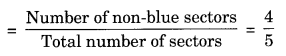Ex 5.3 Class 8 Maths Question 6.
Find the probabilities of the events given in Question 2.
Solution:
Refer to Question 2, we have
(i) (a) Probability of getting a prime number(b) Probability of getting a non-prime number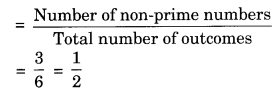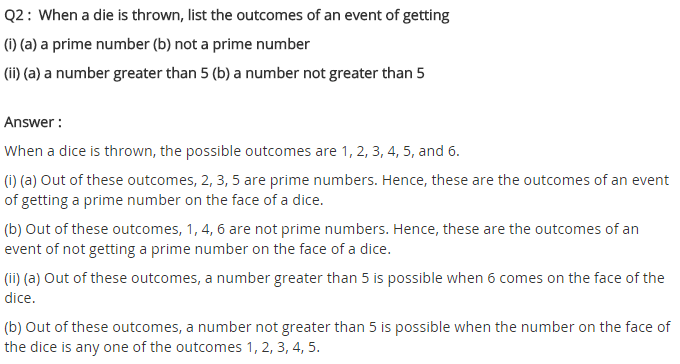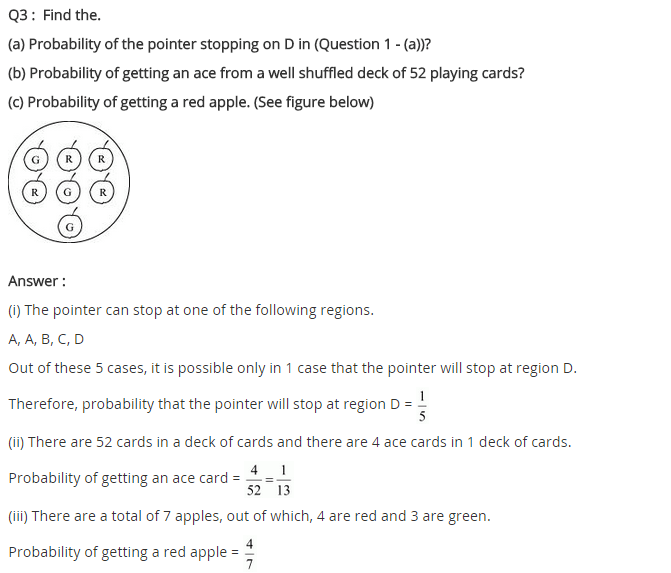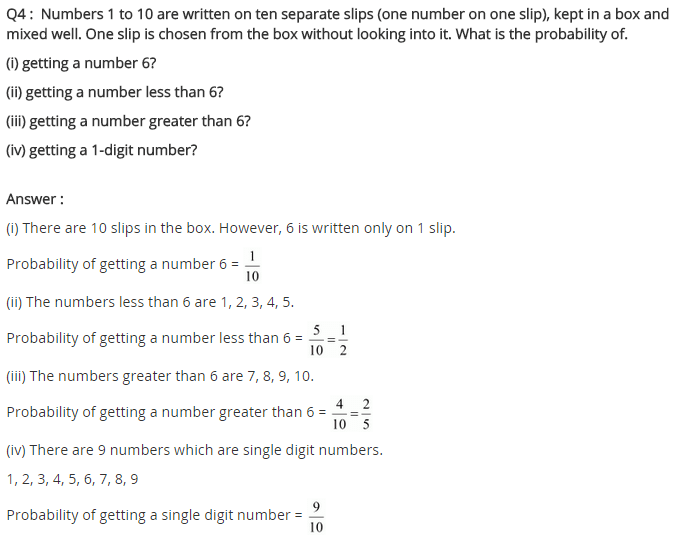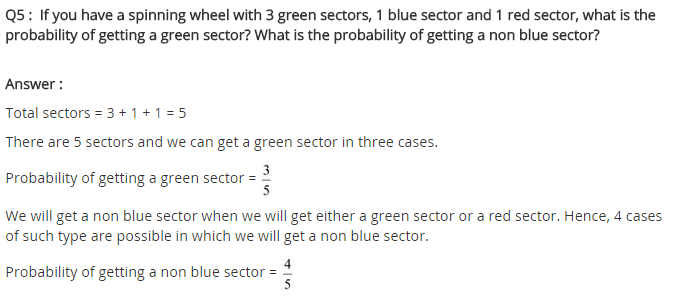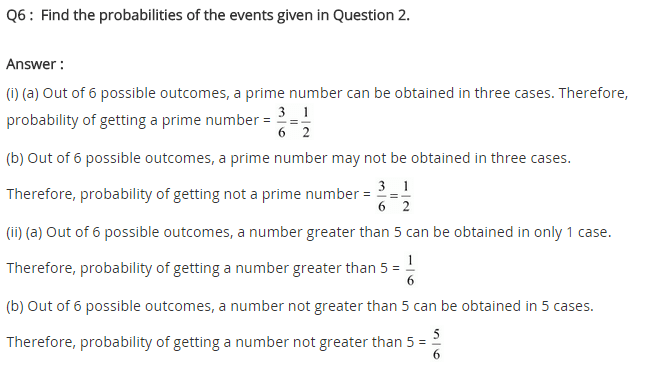## SabDekho

The Complete Educational Website